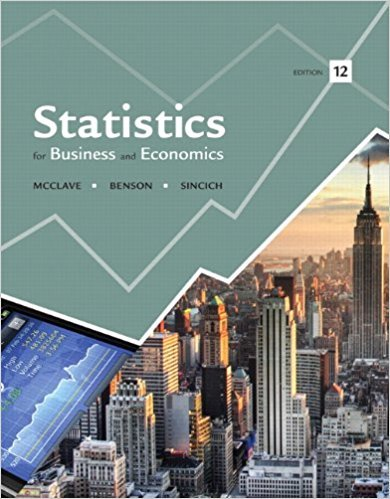×
Get Full Access to Statistics For Business And Economics - 12 Edition - Chapter 9 - Problem 82se
Get Full Access to Statistics For Business And Economics - 12 Edition - Chapter 9 - Problem 82se

×

# What is the difference between a one-way ANOVA and aISBN: 9780321826237 51

## Solution for problem 82SE Chapter 9

Statistics for Business and Economics | 12th Edition

• Textbook Solutions
• 2901 Step-by-step solutions solved by professors and subject experts
• Get 24/7 help from StudySoup virtual teaching assistantsStatistics for Business and Economics | 12th Edition

4 5 1 406 Reviews
24
2
Problem 82SE

Problem 82SE

What is the difference between a one-way ANOVA and a two-way ANOVA?

Step-by-Step Solution:
Step 1 of 3

Introduction to Chapter 1:  Difference of Squares: a -b =(a-b)(a+b)  Zero Product Property: ab=0; a, b=0 1.1 Linear Equations are first-degree equations in one variable that contain the equality symbol, “=”. Linear equation in the variable x can be expresses in the standard form, ax+b=c, where a ≠, b ∊ ℝ The domain is the largest set of acceptable input values. Of note, the denominator of a fraction cannot be equal to zero. 1.1.1 Solve ax+b=0, solve for x ax+(b-b)=(0-b) 1. Isolate x by subtracting b from both sides. x/a=-b/a 2. Divide both sides by a x=-b/a 1.1.2 Solve 5x-3=2x+8 -2x -2x 1. Subtract 2x from each side 3x-3=8 +3 +3 2. Ad

Step 2 of 3

Step 3 of 3

##### ISBN: 9780321826237

Unlock Textbook Solution